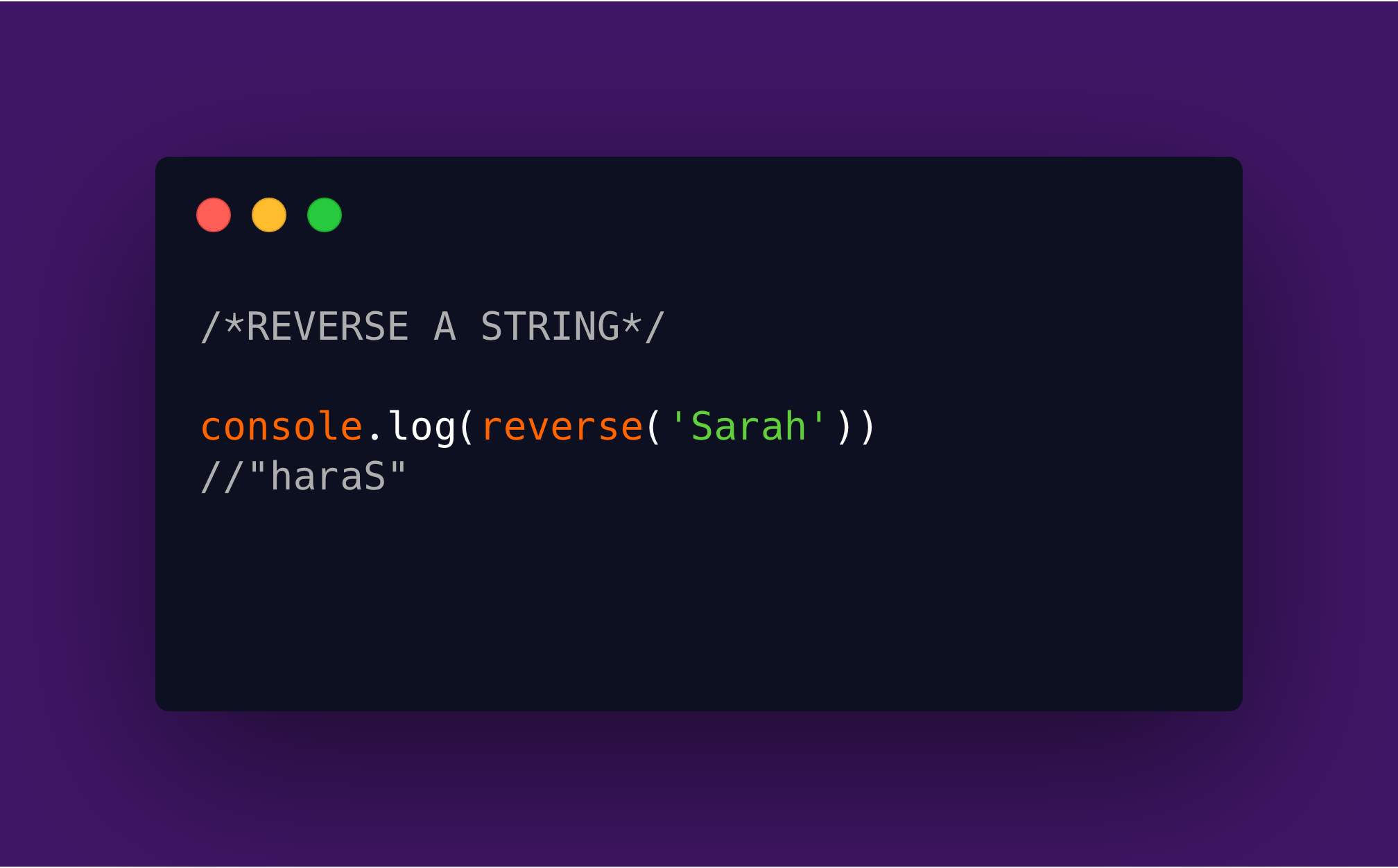# Reverse A String with JavaScript

|The reverse a string problem is a common Algorithm problem. In this article, we will consider four JavaScript solutions to it.

### A Little Background Info

Lately, I have been taking a course on data structures and Algorithms. This is because I realized that I suck at it. This has not always been the case. When I started learning JavaScript, solving algorithm challenges was the fun part for me. I could stay up for hours late at night just trying to solve a challenge. But two years and many frontend projects later, it seems I've forgotten everything I learned. That is why I decided to go back to learn and practice.

A solid knowledge of data structures and algorithms comes with much practice. And what better way to remember what I learn that to write about it. That's why I am presenting to you the first part of a series of articles to come.

Let's delve into our algorithm challenge for today: Reversing a String

###The Problem Reversing a string is, well, reversing a string. Okay, here's the problem statement: Write a function that reverses a string. If you pass "Sarah" to the function, it should return "haraS" and "listen" should become "netsil". Got it?

Now, let's look at four javascript solutions.

###Solution 1. Using the array reverse() method

Thanks to the Array.reverse() method, we can reverse an array without much stress. The reverse() method reverses an array in place. The first array element becomes the last, and the last array element becomes the first.

In our case though, we are working with strings. So this means that we have to convert the string to an array using the split method, reverse it using the reverse method and convert it back to a string using the join method. Here's the code example.

``````function reverseString(string) {
//convert the string to an array
let array = string.split("");

//Use the reverse method
array.reverse()

//Convert it back to a string and return
return array.join("")
}
``````

We can convert this to a one-liner using arrow function and implicit return.

``const reverseString = (string) => string.split("").reverse().join('');``

That's it. One line of goodness. Let's move to the next solution.

###Solution 2: Good Ol' For-loop This is the classic example of reversing through a string. It might be one of the first methods that will come to your mind if you encounter this problem.

What we will do here is to create an empty string that will hold the reversed strng, loop through each character in the string and append it to the beginning of the new string.

``````	function reverse(str) {
let reverseString = "";

for (let character of str) {
reverseString = character + reverseString;
}

return reverseString
}``````

###Solution 3 - Using the Array.reduce() method

The reduce() method executes a reducer function (that you provide) on each element of the array, resulting in a single output value. You can read about it if you are not familiar with it.

To use the reduce method, we need to convert our string to an array. Next, we use the reduce method to convert it to a string. The reducer in this case appends each character of the string to the accumulator which in the code below is `reversed`.

``````function reverseString(string) {
//convert the string to an array
const array = string.split('');

//use the reduce method to convert the array to a reversed string
const reversedString = array.reduce((reversed, character) => {
return character + reversed
}, '')

return reversedString
}``````

This function above can further be reduced to :

``````const reverseString = (string) => {
return string.split('').reduce((reversed, character) => character + reversed, '')
}``````

Making it a one-liner and shortening the name of the variables used in the reduce method.

``const reverseString = (string) => string.split('').reduce((rev, char) => char + rev, '')``

### Solution 4 - Using recursion

Are you a friend of recursion? If not, let me introduce you to it. Recursion is a way of solving a problem by using a function that calls itself. Each time the function calls itself, it reduces the problem into subproblems. This recursive call continues until a point is reached where the subproblem can be called without further recursion.

An important part of a recursive function is a base case. This is the condition(s) where the recursive call terminates to ensure it does not result to an infinite loop. You can find more explanations here.

Back to reversing a string. In this case, our base case is when the string is empty. We use the string.substring() method to get remove the first character in the string and pass the other characters to the function. Then we append the first character to our return statement as seen in the code below.

``````function reverse(string){
//base case: if str is empty return str
if(string === ""){
return string
} else{
return reverse(string.substring(1)) + string
}
}``````

We can use a ternary operator instead of if-else statements.

``````function reverse(string){
return string ? reverse(string.substring(1)) + string : string
}``````

So there you have it, four cools ways to reverse a string in JavaScript.

Got any question or feedback reach out to me via my contact form.

Go follow me on Instagram where I post software development tips and advice.

Be the first to know when I publish a new article. Fill the form below. I promise not to spam.

← All Articles